100010041100400×150×2701950×350×950400×453×125300×390×14001000×410×1090850×850×2000300×350×1380350×420×1200500×510×1400560×470×1838300×95×410620×440×1800330×330×1070380×550×1830400×360×1445420×460×1200431×310×133550×500×15001000×410×11701020×470×17501500×700×1500325×310×980370×370×1200410×160×480420×197×190430×320×1030470×180×420475×140×373500×470×1150/1350/1550/1750650×600×1900900×1150×2000940×1140×20001600×420×8602100×1100×1200230×330×485238×360×580350×155×470350×345×1200357×125×497400×200×510400×200×710400×407×1580425×320×1030450×550×1450480×445×1400500×280×650500×480×1500520×530×1580537×386×153mm560×470×1560600×450×1530620×590×1760800×380×860800×600×17001040×420×12001100×420×12601100×420×14501100×420×8601100×455×18001200×420×10301200×420×10501200×420×8401200×450×15301400×410×11701400×410×15501500×420×12601500×530×17601600×420×10501600×420×12501600×450×15301800×420×10501900×1100×12001900×450×10301900×450×930285×305×880295×90×385300×135×390300×456×30310×76×398315×90×445325×405×110330×170×425350×350×300350×370×1145360×190×450365×430×1302380×420×1220383×298400×390×1580400×400×900400×415×1490400×425×1310407×135×431418×430420×230×370425×212×465430×320×1230430×320×1280430×400×1400440×450×1470450×340×13004580×1600×37804600×3100×7500480×165×382480×530×1450490×160×390520×420×1500520×450×1420530×480×4355330×1530×4370540×530×1770550×400×1720550×520×1500560×560×1490560×650×1650580×420×1450600×500×1100600×550×1960600×600×1700600×600×175060×30×1700640×315×4456500×5200×114006500×5300×12070650×570×1780650×600×1700650×750×1800655×455×18000680×470×1750760×420×1200800×400×1030800×420×1450800×420×1500800×420×830800×500×1550800×600×1900830×440×1850900×300×300900×380×1050900×440×1750900×450×1530900×530×1920950×860×1850950×860×2030995×740×375Φ205×43210000×600×112001000×410×15501000×420×14001000×420×14201020×470×14201050×460×12701060×420×13801080×410×9301080×450×12601100×390×11801100×390×12601100×420×10501100×420×12301100×420×13001100×420×13801100×450×1400110×42×1051150×470×14201160×520×18001200×420×14001200×420×14201200×420×8501200×450×10001200×600×1900120×410×8701230×410×10801250×410×10801250×410×11001250×410×8701400×410×10901400×450×14001500×390×11801500×420×12301500×420×13801500×420×14001500×420×14501500×500×13851600×460×1270160×42×1051800×420×14901800×420×8501880×410×10801880×410×11001910×410×10801910×410×870220×220×600230×9502500×1100×120029×23×48300×330×860300×600×1200300×600×600310×85×435320×320×1240320×350×1250325×85×405335×140×450350×150×450350×360×1100350×400×1240350×450×150360×360×1070360×360×1170360×365×400365×392×1300366×380×130036×36.5×40cm370×160×510370×170×400370×400×1190380×190×470380×290×1100380×460×1250390×270×860400×400×1200400×400×995400×420×1520400×475×1420410×240×520410×240×720410×330×147042.5×21.2×46.5cm420×350×550421×452×1455425×320×1250425×32×12542×23×37cm430×320×104430×320×1040430×320×1050430×320×1150430×330×1400430×330×1420438×33043×55×13243×55×134.5450×280×380450×300×540450×300×550450×300×750450×330×1080450×340×1280450×340×950450×350×1170450×400×1550450×410×1450450×410×1500450×450×1480450×480×1330460×140×360460×145×380460×150×420460×240×410460×380×1350470×116×375470×498×1231475×145×386475×300×1109475×300×6494800×2000×3000480×193×433480×380×1300490×388×228490×388×228mm490×395×490500×330×1050500×350×1120500×390×1180500×390×1260500×420×1380500×440×1570500×530×1450500×530×1650503×495×1657505×36×165510×590×860mm530×1720×470537×386×153550×420×1400550×450×1500550×500×1600550×500×1800550×510×1770550×520×1600550×520×1800550×530×1600550×530×1800560×380×800570×500×1630580×420×1100

0.10.60.811.251.622.12.234.567912600W3KW12KW1250W6KW9KW100W2KV

220V220VAC 50Hz/380VAC 50Hz380V220v 50HZ/380v 50HZ220V/50HZ380V/50Hz220V 50HZ380vAC100-240/50-60Hz

202020192018201713%
2020/10/21
/13%
2020/09/26
/13%
2020/09/25
/13%
2020/09/24
/13%
2020/09/21
/13%
2020/08/24
/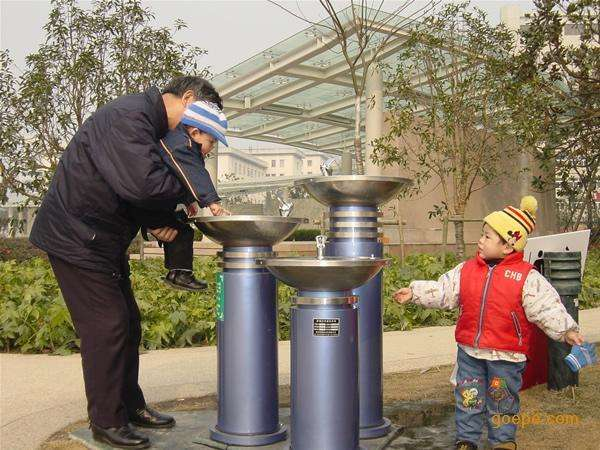13%
2020/10/1813%
2020/10/1813%
2020/10/1813%
2020/10/18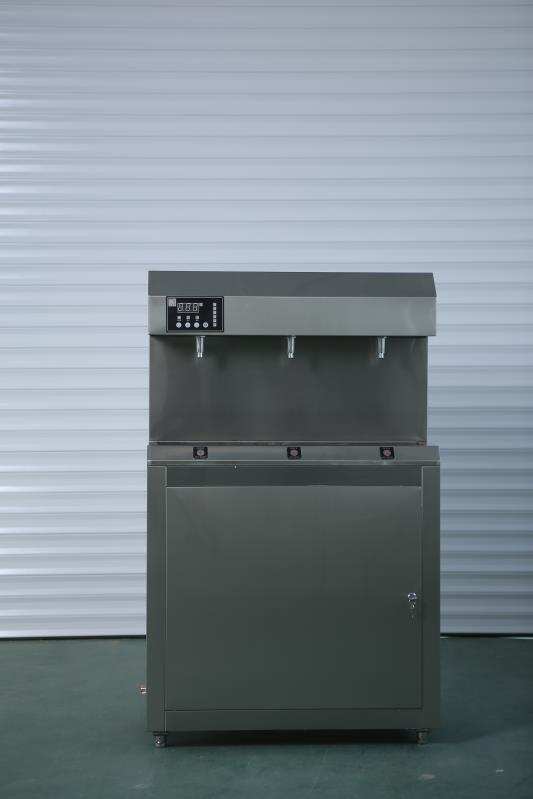13%
2020/10/1813%
2020/10/1813%
2020/10/1813%
2020/10/1813%
2020/10/18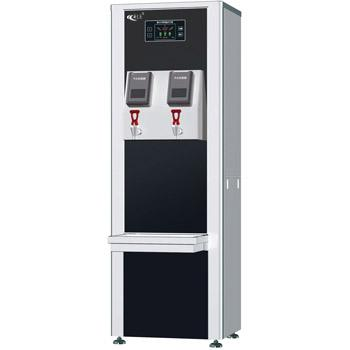13%
2020/10/1813%
2020/10/18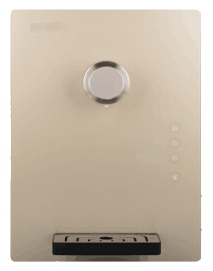13%
2020/10/18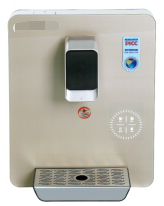13%
2020/10/1813%
2020/10/18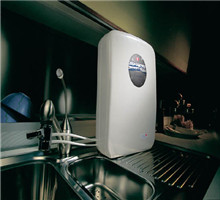13%
2020/10/1813%
2020/10/1813%
2020/10/1813%
2020/10/1813%
2020/10/1813%
2020/10/1813%
2020/10/1813%
2020/10/1813%
2020/10/1813%
2020/10/18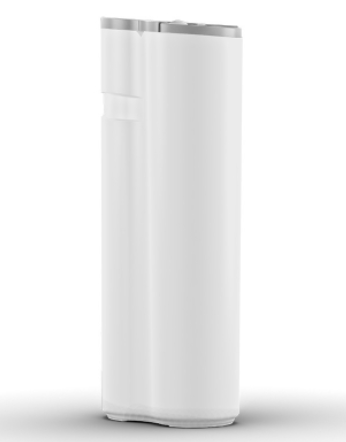13%
2020/10/1813%
2020/10/18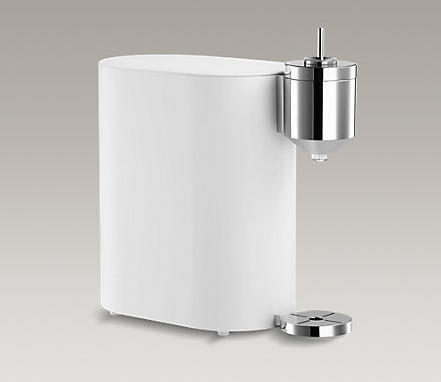13%
2020/10/18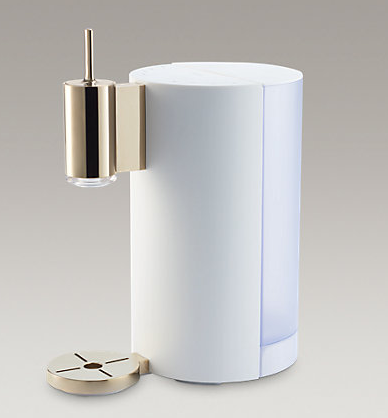13%
2020/10/1813%
2020/10/1813%
2020/10/18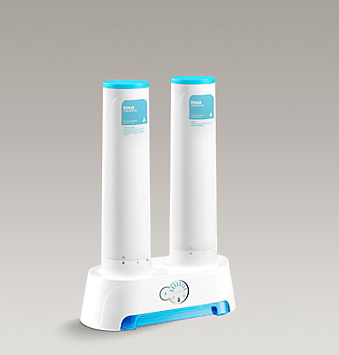13%
2020/10/1813%
2020/10/1813%
2020/10/18

• 1
• 2
• 3
• 4
• 5
• 6
• 23订阅中心广联达大数据